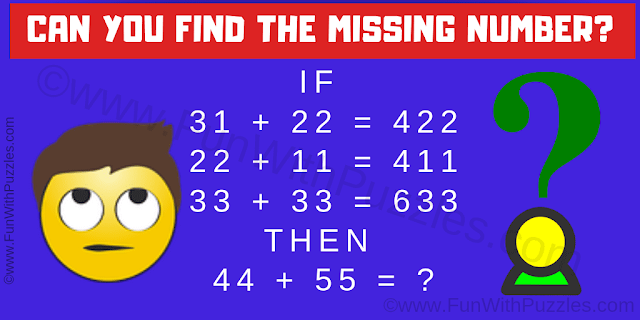This is a logical easy math question for kids. This math logic puzzle will test your mathematical skills. You are shown the number equation in this logic math question and your challenge is to decipher the logical reasoning in these equations. Apply the same logic to the last number equation and then find the value of the missing number which will replace the question mark.Can you solve this easy logic math question?

The answer to this "Easy Logic Math Question", can be viewed by clicking on the answer button.